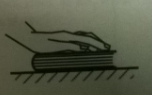# Problem:  Consider a book on top of a level table while the book is being pressed straight down by a hand.i. In the space below, draw a free-body diagram for the book. Label the forces.ii. How do the forces exerted on the book in this case compare to the forces exerted on the book when the hand is not pushing? List any forces that are the same (i.e. same type of force, same direction, and same magnitude) in both cases. Make a separate list of forces that change (or are not present) when the hand is pressing down on the book.iii. Is the magnitude of the weight equal to the magnitude of the normal force exerted by the table on the book in both cases? How can you tell?

###### FREE Expert Solution

In free body diagrams, we draw a dot to represent the object.

All forces are represented by vectors. The tail of the vectors touches the dot.

(i)

The normal force is directed up.

The weight is directed down.

The force from the hand is directed downward.

89% (447 ratings)###### Problem Details

Consider a book on top of a level table while the book is being pressed straight down by a hand.i. In the space below, draw a free-body diagram for the book. Label the forces.

ii. How do the forces exerted on the book in this case compare to the forces exerted on the book when the hand is not pushing? List any forces that are the same (i.e. same type of force, same direction, and same magnitude) in both cases. Make a separate list of forces that change (or are not present) when the hand is pressing down on the book.

iii. Is the magnitude of the weight equal to the magnitude of the normal force exerted by the table on the book in both cases? How can you tell?